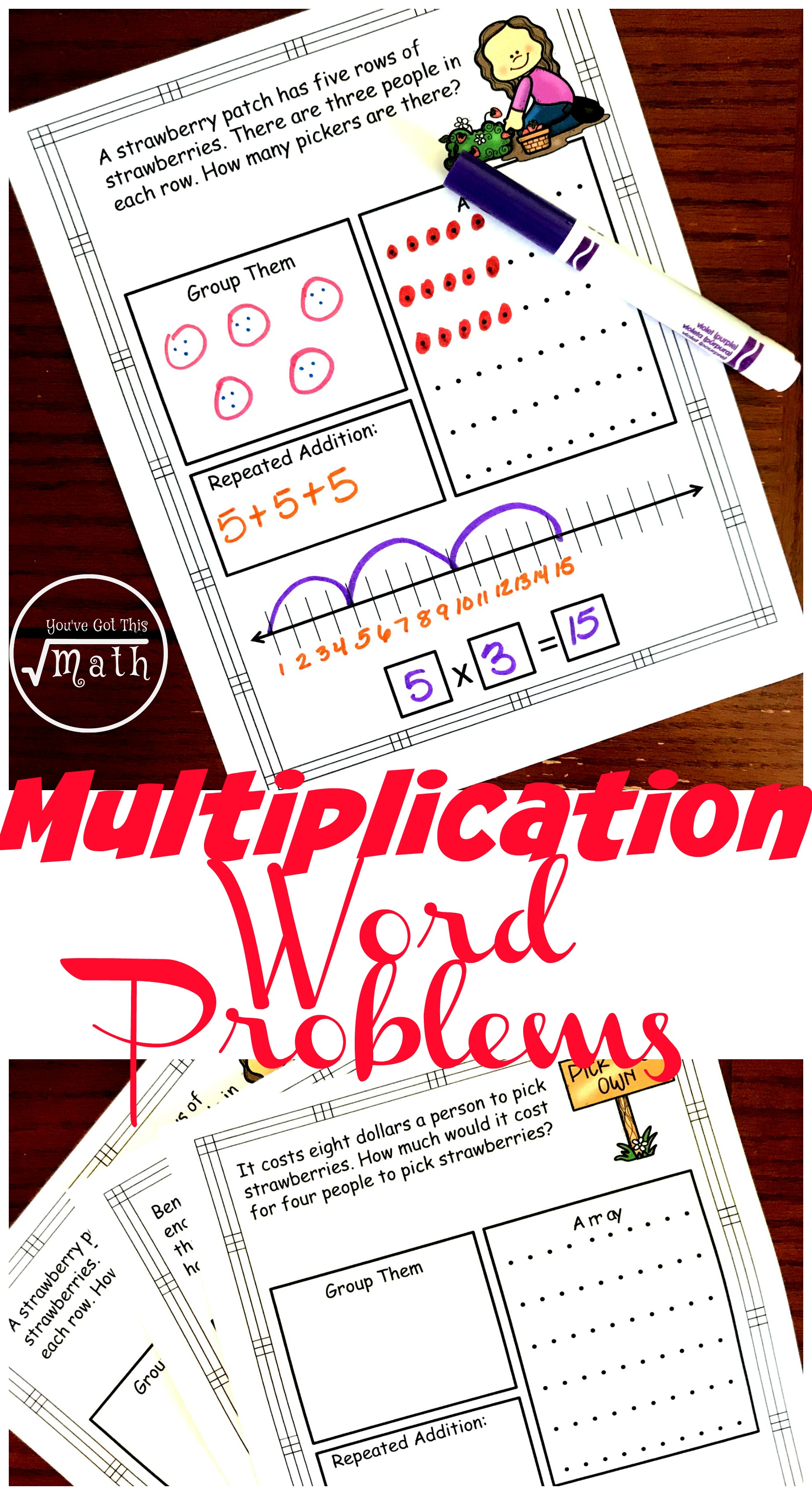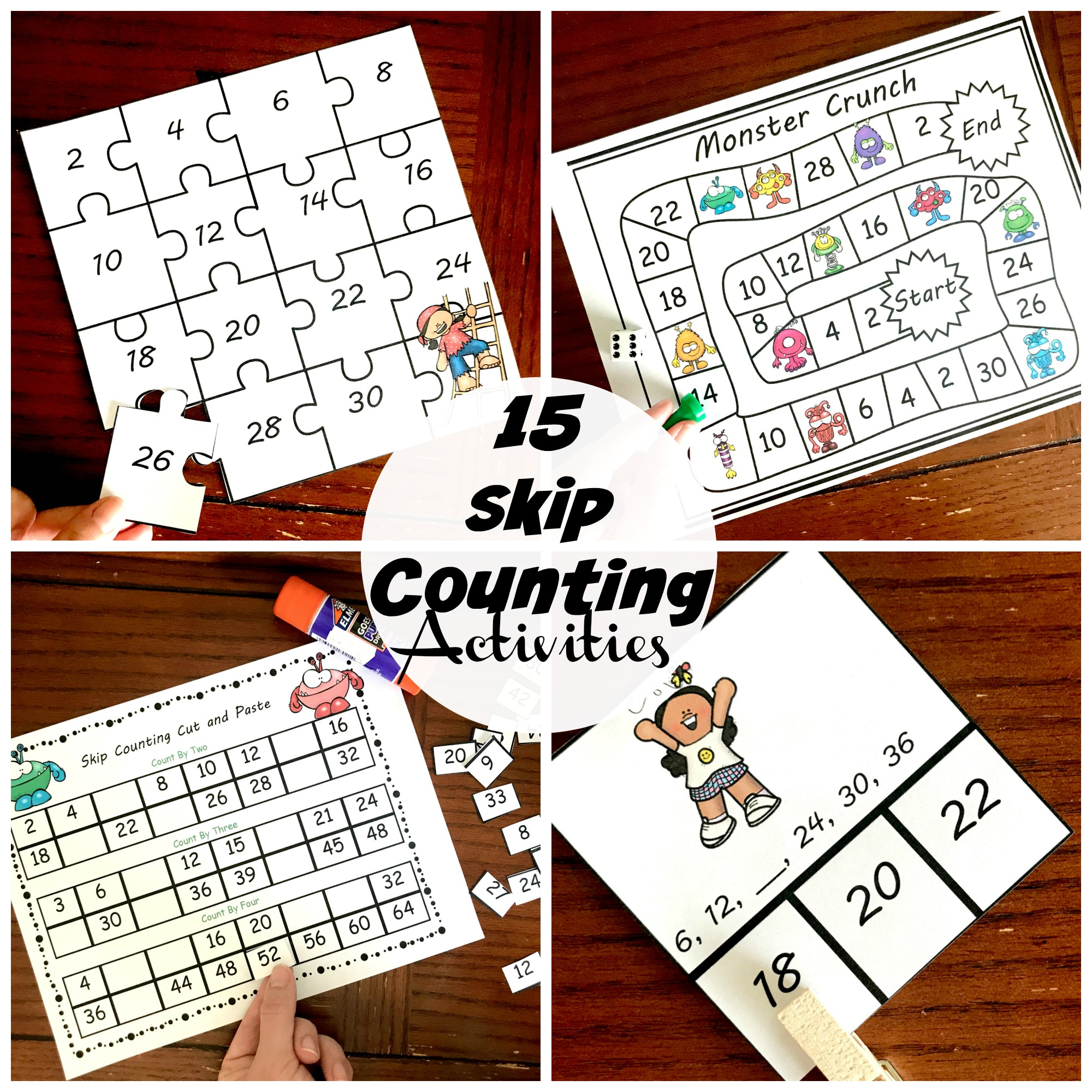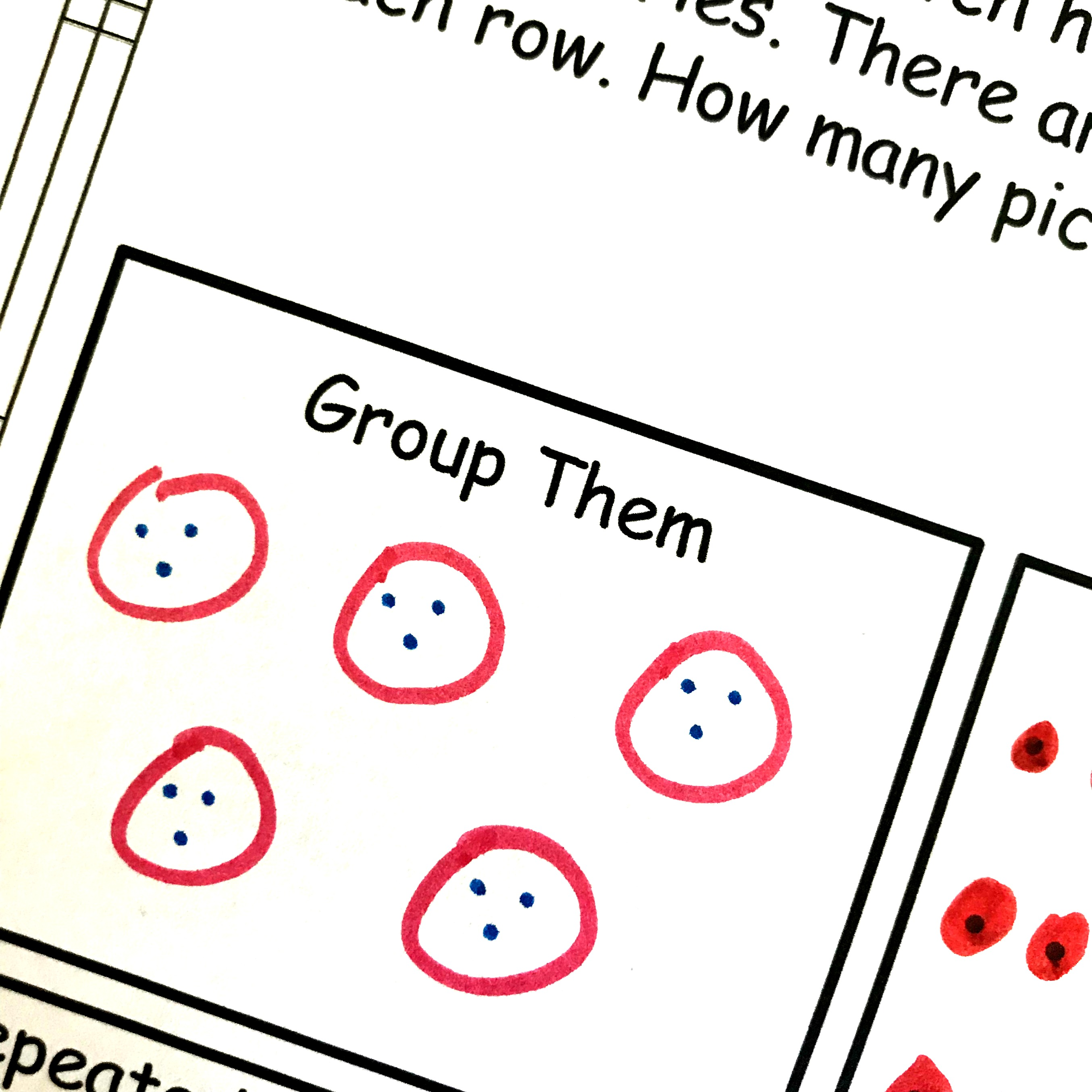Home » Math » FREE Interactive Multiplication Word Problems Worksheets

FREE Interactive Multiplication Word Problems Worksheets

These FREE multiplication word problems worksheets get children solving multiplication word problems through arrays, equations, repeated addition and more.Word problems! They were the bain of my existence as a child.

But now I totally understand the importance of them. It is the only math I do in the real world.

Flooring: I have a 2,000 square foot house and this flooring is 1.25 and this is 1.99. I love the 1.99 one but can I afford it??

Pool Party: There is a pool party at a park and it is \$7 dollars a person. How much am I going to spend for my family of six to go, and is it worth that cost?

Candy: There are 12 servings and each serving is 3 pieces of candy. Will that be enough for all the Valentine’s Day cards my four kids are making???

All of those are problems I have recently solved, and all of them deal with multiplication. Our children need to be comfortable solving word problems because that is the math they will need!

So today, I have five hands-on multiplication problems for your kiddos to work through! These FREE multiplication word problems worksheets are the perfect way to introduce multiplication word problems.

If your children don’t really know their facts, a good place to start is with skip counting.

Check out a few of our activities for skip counting here.Prep-Work

These worksheets are no-prep sheets.

1. First, print of the pages you need.
2. Then grab some pencils and markers and you are ready to go.

Multiplication Word Problems Worksheets

This packet has five-word problems and they each as the children to solve the problem in five different ways.

Group ThemWe begin by having children create circles and placing dots in each circle.

In this example, we see that I have five rows of strawberries. So I begin by drawing three circles. Since there are three people in each row, I place three dots in each circle. Now I can skip count, or add up the dots.

3 + 3 + 3 + 3 + 3 = 15

ArraysArrays are a wonderful way to teach multiplication. It reinforces the idea of repeated addition and lays the foundation for area.

My word problem tells me that I have five rows of strawberries, so I begin by coloring in five dots! Next, we notice that there are three people in each row. So I’m going to have to color in five dots on each row two more times (I’ve already done it once, and I only need a total of three.)

Now we can skip count or add to see what my answer is.

5, 10, 15

5 x 3 = 15

We have pretty much already done this, but now it is time to write it out in an equation.

There are five rows, with three people in each row. So I can say 5 + 5 + 5 = 15

Number LinesNumber lines once again drive home the point that multiplication is repeated addition.

Here we start at one on the number line, and then we hop up the number line based on the problem. So we know that we have five rows, so each time we hop we are going up five. How many times do we have to go up five spaces? Yes! Three hops….and of course we land on fifteen.

In the last two problems, the answers are a little higher. I don’t want to write numbers to 40, and neither do our kiddos. So for these problems, the children can just write at the bottom the numbers they will use.

In this example, the child is supposed to show 8 x 4. So they wrote the multiples of 8 on the number line and then showed four hops.

Equations:

Finally, we come to the equation….and at this point, they know what numbers they have worked with so it is quite easy.

5 x 3 = 15

I hope this helps your children as the conquer the mysterious and fun world of word problems.

You’ve Got This,

Rachel

You May Also Like:

Our division word problemsFive Multiplication Activites in one easy download: